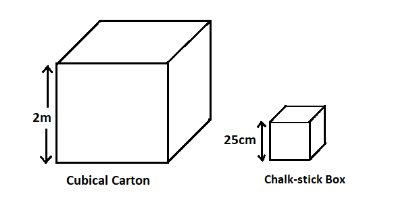The length of a cubical carton is 2 m. How many cubical chalk-stick boxes with length 25 cm can be packed in it.

# The length of a cubical carton is 2 m. How many cubical chalk-stick boxes with length 25 cm can be packed in it.

1. A
154
2. B
150
3. C
512
4. D
148

Fill Out the Form for Expert Academic Guidance!l

+91

Live ClassesBooksTest SeriesSelf Learning

Verify OTP Code (required)

### Solution:

We are given the length of a cubical carton and a cubical chalk-stick box as 2 m and 25 cm respectively. We have to find the no. of chalk-stick boxes that can be packed in the carton.Volume of the carton with length 2 m is1 metre is equal to 100 centimetres.
1 cubic metre is equal tocentimetres.
This meAns:Volume of the chalk-stick box with length 25 cm isTotal no. of chalk-stick boxes that can be packed in the carton is n.Therefore, 512 cubical chalk-stick boxes can be packed in the cubicle cartoon.
So, the correct
Ans: is Option 3.

## Related content

 Area of Square Area of Isosceles Triangle Pythagoras Theorem Triangle Formula Perimeter of Triangle Formula Area Formulae Volume of Cone Formula Matrices and Determinants_mathematics Critical Points Solved Examples Type of relations_mathematics+91

Live ClassesBooksTest SeriesSelf Learning

Verify OTP Code (required)